Anomaly detection (or outlier detection) is one of the many tasks that keep Data Scientists awake at night when then need to satisfy the business needs. No matter whether the data in well structured (like user events) or unstructured (like text or images), there are plenty of methods that allow to tackle this problem and it’s diffictult to choose one. Sometimes, it’s even difficult to properly define, what an anomaly is - but that’s not the part of this post’s main focus. Some of the most popular methods for anomaly detection are:

• statistical methods - like three-sigma rule and regression models
• classical machine learning methods - like one-class SVM, Isolation Forests or clustering-based methods (e.g. kNN)
• deep learning methods - like autoencoders and deep forecasting models (e.g. LSTMs for time series)

In this post I will focus on a single method called Robust Subspace Recovery Layer for Unsupervised Anomaly Detection (https://arxiv.org/abs/1904.00152) which is an extension of the autoencoder-based unsupervised anomnaly detection method for data of any domain.

## TL;DR

Implementation of RSR Layer and RSR Autoencoder (RSRAE) in PyTorch. Link to Jupyter Notebook / Google Colab at the bottom.

Note: This post and my implementation does not reproduce RSR Layer paper. I only explain the general idea and provide minimal working code as a form of proof-of-concept.

## Idea behind Robust Space Recovery Layer

Autoencoders were used for the anomaly detection much earlier than this (RSR) paper appeared. To use autoencoder in a setting of anomaly detection, one treats “normal” examples (inliers) as the ones that have low reconstruction error and other which have high reconstruction error are treated as outliers. Although the idea is fairly easy to understand and implement, the problem lies in the fact that in order for autoencoder to work properly in this setting (i.e. to detect the outliers) the training data should be clean (or have really small fraction of the outliers). In the real world it’s highly unlikely for the input data to be free of any anomalies and even if Data Scientists could label and filter the data (given they know what an anomaly in the given dataset is) it simply won’t scale.

The Robust Subspace Recovery Layer paper tries to adress this issue by incorporating the RSR Layer into autoencoders. It’s clearly stated that this method does not compete with semi-supervised or supervised anomaly detection setting, but shows its strenght in the unsupervised setting.

### What is the RSR Layer?

The RSR Layer itself is just an additional layer in the nerual network with specific properties. It maps one representation (embedding obtained from the encoder) into another low-dimensional representation that is outlier-robust.

Let’s say that D (upper case D) is the dimension of the embedding from the encoder. The assumption in the paper is that the “normal” data lies within d-dimensional (lower case d) manifold (“subspace”) of the original embedding, which means that d < D.

Please refer to the paper to obtain more details and the full explanation and analysis. At this point you should know enough to proceed to the implementation section.

## How to implement the RSR Layer in PyTorch?

As RSR Layer is just a linear mapping, there are only a few things needed:

1. The dimension D from the encoder’s output.
2. The dimension d which is a direct property of the RSR Layer (and probably requires a hyperparamer search to set it properly).
3. Proper initialization of the linear mapping weights (the optimization goal of the RSR Autoencoder enforces that, so it’s better to start with a set of weights that already fulfill this constraint).

The implementation of the layer itself is straightforward.

``````import torch
from torch import nn
import torch.nn.functional as F
``````
``````class RSRLayer(nn.Module):
def __init__(self, d:int, D: int):
super().__init__()
self.d = d
self.D = D
self.A = nn.Parameter(torch.nn.init.orthogonal_(torch.empty(d, D)))

def forward(self, z):
# z is the output from the encoder
z_hat = self.A @ z.view(z.size(0), self.D, 1)
return z_hat.squeeze(2)
``````

The next piece to obtain RSR Autoencoder in PyTorch is to implement RSR Loss as per paper’s equation (4):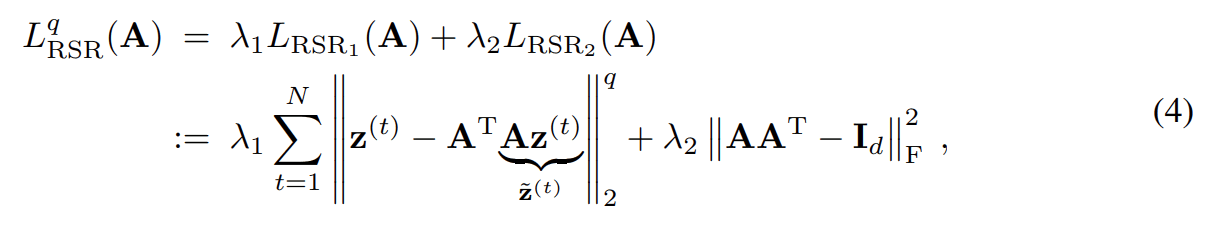The first term enforces the RSR Layer projection to be robust and the second term enforces the projection to be orthogonal. Refer to the paper for the motivation behind this loss as it it out of scope for this blog post.

Nearly op-for-op (math-to-PyTorch) implementation of this loss looks like this:

``````class RSRLoss(nn.Module):
def __init__(self, lambda1, lambda2, d, D):
super().__init__()
self.lambda1 = lambda1
self.lambda2 = lambda2
self.d = d
self.D = D
self.register_buffer(
"Id", torch.eye(d)
)

def forward(self, z, A):
z_hat = A @ z.view(z.size(0), self.D, 1)
AtAz = (A.T @ z_hat).squeeze(2)
term1 = torch.sum(
torch.norm(z - AtAz, p=2)
)

term2 = torch.norm(
A @ A.T - self.Id, p=2
) ** 2

return self.lambda1 * term1 + self.lambda2 * term2
``````

Lastly, the standard L1/L2 reconstruction loss is replaced by the L2,p-norm based loss (again, the paper relies on “more robustness”, wiki: https://en.wikipedia.org/wiki/Matrix_norm#L2,1_and_Lp,q_norms). In practice, the paper uses L2,1 version.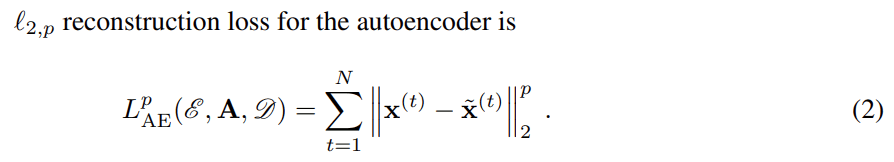``````class L2p_Loss(nn.Module):
def __init__(self, p=1.0):
super().__init__()
self.p = p

def forward(self, y_hat, y):
torch.pow(
torch.norm(y - y_hat, p=2), self.p
)
)
``````

## RSR Auto Encoder in PyTorch

In order to see the RSR Layer in practice, the RSR Autoencoder needs to be implemented.

Important
The following RSR Autoencoder implementation is only a minimal, working proof of concept. As I’ve stated earlier, the intent of this blog post is to implement the RSR Layers and play around with them, not reach the SOTA or reproduce the paper’s results. Feel free to use my code and plug-in your encoder and decoder networks.

``````class RSRAutoEncoder(nn.Module):
def __init__(self, input_dim, d, D):
super().__init__()
self.encoder = nn.Sequential(
nn.Linear(input_dim, input_dim // 2),
nn.LeakyReLU(),
nn.Linear(input_dim // 2, input_dim // 4),
nn.LeakyReLU(),
nn.Linear(input_dim // 4, D)
)

self.rsr = RSRLayer(d, D)

self.decoder = nn.Sequential(
nn.Linear(d, D),
nn.LeakyReLU(),
nn.Linear(D, input_dim // 2),
nn.LeakyReLU(),
nn.Linear(input_dim // 2, input_dim)
)

def forward(self, x):
enc = self.encoder(x) # obtain the embedding from the encoder
latent = self.rsr(enc) # RSR manifold
dec = self.decoder(latent) # obtain the representation in the input space
return enc, dec, latent, self.rsr.A
``````

## Training the autoencoder with RSR Layer in PyTorch (& PyTorch Lightning)

As usual, I use PyTorch Lightning to skip the training boilerplate.

``````import pytorch_lightning as pl
pl.seed_everything(666)
``````

### Corrupted MNIST dataset (with outliers)

For this proof-of-concept I will be using a corrupted version of MNIST dataset, that contains single class as “normal” data and a few other, that will be treated as outliers.

``````from torchvision.datasets import MNIST
from torchvision.transforms import ToTensor
# https://github.com/pytorch/vision/issues/1938#issuecomment-594623431
from six.moves import urllib
opener = urllib.request.build_opener()
urllib.request.install_opener(opener)

``````
``````class RSRDs(torch.utils.data.Dataset):
def __init__(self, target_class, other_classes, n_examples_per_other):
super().__init__()
self.target_indices = (self.mnist.targets == target_class).nonzero().flatten()

other = []
for other_class in other_classes:
other.extend(
(self.mnist.targets == other_class).nonzero().flatten()[:n_examples_per_other]
)
self.other_indices = torch.tensor(other)
self.all_indices = torch.cat([self.other_indices, self.target_indices])
print(f"Targets: {self.target_indices.size(0)}")
print(f"Others : {self.other_indices.size(0)}")

def __getitem__(self, idx):
actual_idx = self.all_indices[idx].item()
return self.mnist[actual_idx]

def __len__(self):
return self.all_indices.size(0)
``````
``````ds = RSRDs(target_class=4, other_classes=(0, 1, 2, 8), n_examples_per_other=100)
``````
``````Targets: 5842
Others : 400
``````
``````ds.shape, ds
``````
``````(torch.Size([1, 28, 28]), 0)
``````

### RSRAutoencoder module in PyTorch Lightning

The following module combines all of the pieces together:

1. RSR Autoencoder with RSR Layer inside,
2. L2p reconstruction loss,
3. RSR Loss.
``````class RSRAE(pl.LightningModule):
def __init__(self, hparams):
super().__init__()
self.hparams = hparams
self.ae = RSRAutoEncoder(
self.hparams.input_dim,
self.hparams.d,
self.hparams.D)
self.reconstruction_loss = L2p_Loss(p=1.0)
self.rsr_loss = RSRLoss(self.hparams.lambda1, self.hparams.lambda2, self.hparams.d, self.hparams.D)

def forward(self, x):
return self.ae(x)

def training_step(self, batch, batch_idx):
X, _ = batch
x = X.view(X.size(0), -1)
enc, dec, latent, A = self.ae(x)

rec_loss = self.reconstruction_loss(torch.sigmoid(dec), x)
rsr_loss = self.rsr_loss(enc, A)
loss = rec_loss + rsr_loss

# log some usefull stuff
self.log("reconstruction_loss", rec_loss.item(), on_step=True, on_epoch=False, prog_bar=True)
self.log("rsr_loss", rsr_loss.item(), on_step=True, on_epoch=False, prog_bar=True)
return {"loss": loss}

def configure_optimizers(self):
# Fast.AI's best practices :)
scheduler = torch.optim.lr_scheduler.OneCycleLR(opt, max_lr=self.hparams.lr,
epochs=self.hparams.epochs,
steps_per_epoch=self.hparams.steps_per_epoch)
return [opt], [{
"scheduler": scheduler,
"interval": "step"
}]

``````
``````dl = DataLoader(ds, batch_size=64, shuffle=True, drop_last=True)
``````
``````hparams = dict(
d=16,
D=128,
input_dim=28*28,
# Peak learning rate
lr=0.01,
# Configuration for the OneCycleLR scheduler
epochs=150,
steps_per_epoch=len(dl),
# lambda coefficients from RSR Loss
lambda1=1.0,
lambda2=1.0,
)
model = RSRAE(hparams)
model
``````
``````RSRAE(
(ae): RSRAutoEncoder(
(encoder): Sequential(
(0): Linear(in_features=784, out_features=392, bias=True)
(1): LeakyReLU(negative_slope=0.01)
(2): Linear(in_features=392, out_features=196, bias=True)
(3): LeakyReLU(negative_slope=0.01)
(4): Linear(in_features=196, out_features=128, bias=True)
)
(rsr): RSRLayer()
(decoder): Sequential(
(0): Linear(in_features=16, out_features=128, bias=True)
(1): LeakyReLU(negative_slope=0.01)
(2): Linear(in_features=128, out_features=392, bias=True)
(3): LeakyReLU(negative_slope=0.01)
(4): Linear(in_features=392, out_features=784, bias=True)
)
)
(reconstruction_loss): L2p_Loss()
(rsr_loss): RSRLoss()
)
``````
``````trainer = pl.Trainer(max_epochs=model.hparams.epochs, gpus=1)
trainer.fit(model, dl)
``````

## Analysing RSR Autoencoder outputs

First, let’s check the general autoencoder reconstuction.

``````model.freeze()
from torchvision.transforms import functional as tvf
from torchvision.utils import make_grid
def reconstruct(x, model):
enc, x_hat, latent, A = model(x.view(1, -1))
x_hat = torch.sigmoid(x_hat)
return tvf.to_pil_image(
make_grid([x_hat.squeeze(0).view(1, 28, 28), x])
)
``````
``````tvf.to_pil_image(
make_grid([tvf.to_tensor(reconstruct(ds[i], model)) for i in torch.randint(0, len(ds), (8, ))], nrow=1)
)
``````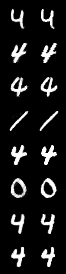Most of the images seem OK to me, given how simple the model is.

Now let’s look at the RSR embedding space projected into 3D using PCA.

``````import pandas as pd
rsr_embeddings = []
classes = []
errors = []
for batch in iter(DataLoader(ds, batch_size=64, shuffle=False)):
X, cl = batch
x = X.view(X.size(0), -1)
enc, x_hat, latent, A = model(x)
rsr_embeddings.append(latent)
classes.extend(cl.numpy())
for i in range(X.size(0)):
rec_error = L2p_Loss()(torch.sigmoid(x_hat[i]).unsqueeze(0), x[i].unsqueeze(0))
errors.append(float(rec_error.numpy()))

all_embs = torch.vstack(rsr_embeddings)
df = pd.DataFrame(all_embs.numpy(), columns=["x", "y", "z"] + [f"dim_{i}" for i in range(hparams["d"] - 3)])
df.loc[:, "class"] = classes
df.loc[:, "errors"] = errors
``````
``````from sklearn.decomposition import PCA
pca = PCA(n_components=3)
rsr_3d = pd.DataFrame(pca.fit_transform(all_embs), columns=["x", "y", "z"])
rsr_3d.loc[:, "class"] = classes
``````
``````import plotly.express as px
import plotly
plotly.offline.init_notebook_mode()
df = df
fig = px.scatter_3d(df, x='x', y='y', z='z',
symbol="class", color="class", opacity=0.95)
fig.update_layout(margin=dict(l=0, r=0, b=0, t=0))
fig.show()
``````

Indeed, most of the 4 (the main class - inliers) reside in the center of the space, with other classes positioned further away from the center.

## Unsupervised anomaly detection using RSR Autoencoder

Now let’s see if it works as the authors of the paper claim - whether the data points with high reconstruction error are really outliers.

``````df.errors.describe()
``````
``````    count    6242.000000
mean        1.551347
std         0.293904
min         0.615430
25%         1.355003
50%         1.512501
75%         1.707990
max         3.462465
Name: errors, dtype: float64
``````
``````df.groupby("class").errors.hist(legend=True, bins=60, figsize=(12, 12))
``````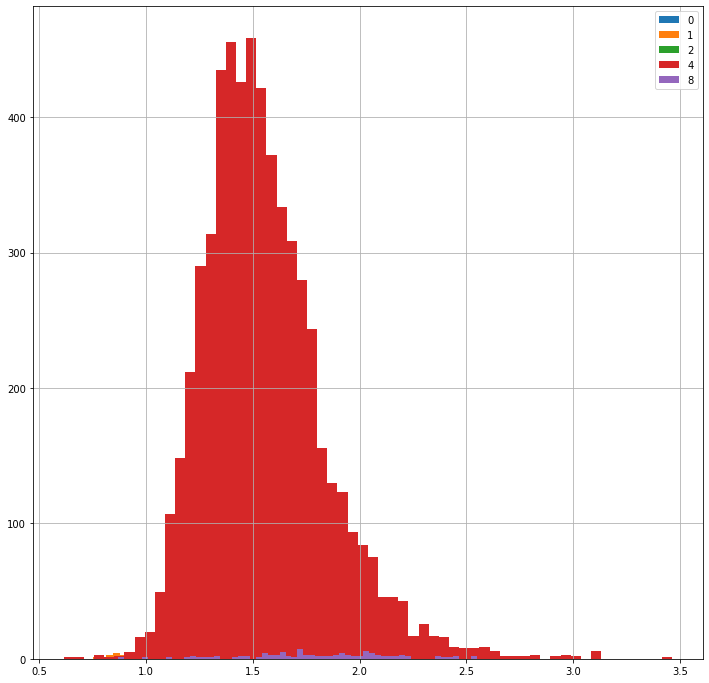The reconstruction loss is centered around `1.5` with a tail spreading up to `3.46`. What are the data points with the largest errors?

``````lowest_mistakes = df.sort_values(by="errors", ascending=True).head(60).loc[:, ["errors", "class"]]
highest_mistakes = df.sort_values(by="errors", ascending=False).head(60).loc[:, ["errors", "class"]]
``````
errors class
5917 3.462465 4
2201 3.120193 4
5834 3.116279 4
6017 3.109386 4
601 3.098328 4
983 3.097286 4
1312 3.091813 4
3304 3.030314 4
6015 2.998559 4
1619 2.974236 4

It seems really odd at first, as I've expected all the non-4 classes to have the largest errors. Let's carefully look at the actual results though:
``````print("Images with the highest reconsturction loss")
tvf.to_pil_image(
make_grid([tvf.to_tensor(reconstruct(ds[i], model)) for i in highest_mistakes.index], nrow=6,
)
``````

Images with the highest reconsturction loss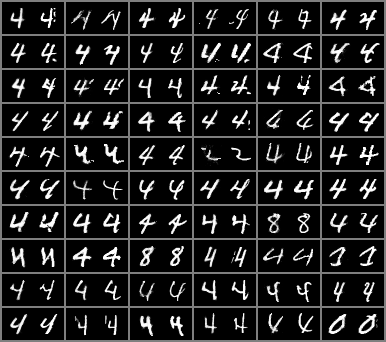``````print("Images with the lowest reconsturction loss")
tvf.to_pil_image(
make_grid([tvf.to_tensor(reconstruct(ds[i], model)) for i in lowest_mistakes.index], nrow=6,
)
``````

Images with the lowest reconsturction loss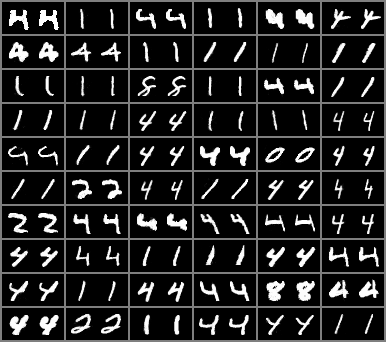Now it’s much clearer - the model found the manifold that separates the images into the “normal” ones - that in this setting seem like more smooth ones with clean curves - and the outliers that are rather sharp, contain some random artifacts (which are anomalies IMHO) and usually seem slightly malformed. Given the model simplicity and the setup, it looks to me like a good starting point for the unsupervised anomaly detection.

## Summary

I hope you find this post useful and it will help you to quickly get started with RSR Layer / RSRAE and unsupervised anomaly detection in PyTorch. Do not hesistate to comment and share!